晓晓 2018-03-15 来源 ：网络 阅读 296 评论 0

1 创建NumericStepper控件

```<mx:NumericStepper minimum="1908" maximum="2000"
x="10" y="10" />
<mx:Label text="年" x="85" y="10" />
<mx:NumericStepper minimum="1" maximum="12"
x="100" y="10" />
<mx:Label text="月" x="160" y="10" />
<mx:NumericStepper minimum="1" maximum="31"
x="175" y="10" />
<mx:Label text="日" x="235" y="10" />```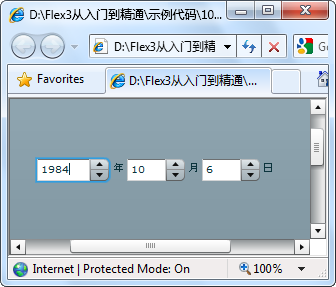2.1使用DateChooser控件实现日期选择

DateChooser控件是一个日历形式的控件，在头部显示了年份和月份的名称，主体部分则是以网格的显示排列了当前年份和月份的所有的天。用户可以从中选择任意的一天。

`<mx:DateChooser x="37" y="10"/>`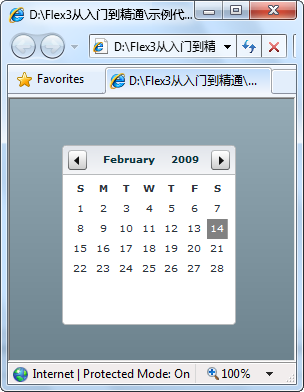2.2 使用DateField控件实现日期选择

DateField控件是一个以文本形式显示的日期控件。在文本框的右侧是一个日历的小图标，当用户单击图表的时候，会弹出一个包含DateChooser控件的窗口。用户选择了一个日期之后，选择的日期会填充到文本框中，同时，此窗口会自动关闭。DateField控件的主要应用在表单等的操作上。

`<mx:DateField x="52" y="46"/>`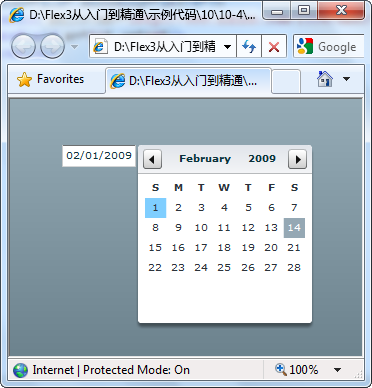2.3 使用日期类

```<?xml version="1.0" encoding="utf-8"?>
layout="absolute" creationComplete="InitApp()">
<mx:Script>
<![CDATA[
// 初始化
private function InitApp():void
{
// 创建日期对象
var date1:Date = new Date(2008, 07, 08);
// 设置默认显示日期
dc1.selectedDate = date1;
}
]]>
</mx:Script>
<mx:DateChooser id="dc1" x="100" y="100" />
</mx:Application>```

2.4 设置DateField控件的日期格式

```<?xml version="1.0" encoding="utf-8"?>
<mx:DateField x="281" y="208" formatString="YYYY-MM-DD" />
</mx:Application>```

2.5 设置DateChooser控件的中文显示

```<?xml version="1.0" encoding="utf-8"?>
<mx:Script>
<![CDATA[
private function InitDataChooser():void
{
// 设置月份名称
var arrMonthName:Array =
["一月", "二月", "三月", "四月", "五月", "六月",
"七月", "八月", "九月", "十月", "十一月", "十二月"];
// 设置周名称
var arrWeekName:Array = ["星期日", "星期一", "星期二",
"星期三", "星期四", "星期五", "星期六"];
dc1.monthNames = arrMonthName;
dc1.dayNames = arrWeekName;
}
]]>
</mx:Script>
<mx:DateChooser id="dc1" x="151" y="86" creationComplete="InitDataChooser()"/>
</mx:Application>```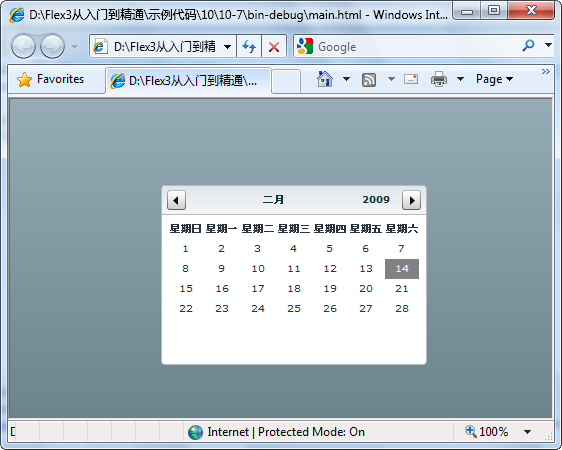三、 加载外部SWF文件

```<?xml version="1.0" encoding="utf-8"?>
</mx:Application>```

3.2 与已加载的SWF文件交互

1．访问已加载的SWF文件中的控件

`lbl.text = loadedSM.application["lblShow"].text;`

2．访问已加载的SWF文件中的变量

```loadedSM = SystemManager(myLoader.content);

3．访问已加载的SWF文件的方法

```loadedSM = SystemManager(myLoader.content);
main.mxml
<?xml version="1.0" encoding="utf-8"?>
<mx:Script>
<![CDATA[
import mx.managers.SystemManager;
// 初始化SWF文件，并把其内容赋给 SystemManager 对象
private function InitSWFApp():void
{
}
// 获取SWF文件中 Label 控件的 text 属性
private function GetSWFLabel():void
{
}
// 修改SWF文件中 Label 控件的 text属性
{
}
]]>
</mx:Script>
<mx:Label id="lbl" x="203" y="62"/>
creationComplete="InitSWFApp();"/>
<mx:Button click="GetSWFLabel();" x="10" y="60" label="获取SWF文件中的Label控件"/>
</mx:Application>
ChildApp.mxml
<?xml version="1.0" encoding="utf-8"?>
<mx:Label id="lblShow" text="这是已加载的SWF文件中的控件" fontSize="20" x="1" y="0"/>
</mx:Application>```

`<mx:Image x="10" y="10" source="@Embed(source='assets/test.jpg')"/>`

4.2 加载图像

```<?xml version="1.0" encoding="utf-8"?>
<mx:Script>
<![CDATA[
private function InitImage():void
{
// 加载图片资源
}
]]>
</mx:Script>
<mx:Image id="img" x="10" y="10" creationComplete="InitImage();"/>
</mx:Application>```

5.1创建ColorPicker控件

`<mx:ColorPicker x="10" y="10"/>`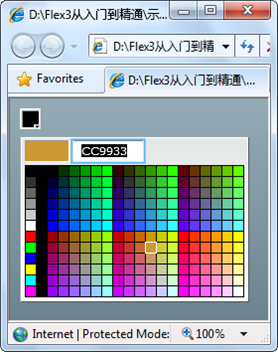5.2 自定义面板显示的颜色

```<?xml version="1.0" encoding="utf-8"?>
<mx:Script>
<![CDATA[
[Bindable]
// 创建数组，填充各种颜色的RGB值
public var simpleDP:Array = ['0x000000', '0xFF0000', '0xFF8800',
'0xFFFF00', '0x88FF00', '0x00FF00', '0x00FF88', '0x00FFFF',
'0x0088FF', '0x0000FF', '0x8800FF', '0xFF00FF', '0xFFFFFF'];
]]>
</mx:Script>
<mx:ColorPicker x="10" y="10" dataProvider="{simpleDP}" />
</mx:Application>```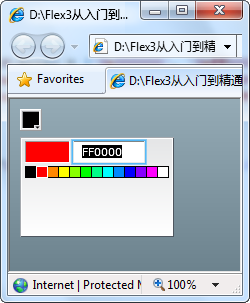5.3 自定义面板显示的标签

```<?xml version="1.0" encoding="utf-8"?>
<mx:Script>
<![CDATA[
import mx.events.ColorPickerEvent;
import mx.events.DropdownEvent;
// 创建数组，并填充颜色相关数据
[Bindable]
public var complexDPArray:Array = [
{label:"黄色", color:"0xFFFF00",
descript:"黄色"},
{label:"粉色", color:"0xFF66CC",
descript:"粉色"},
{label:"深红色", color:"0x990000",
descript:"深红色"},
{label:"蓝色", color:"0x000066",
descript:"蓝色"},
{label:"深绿色", color:"0x006600",
descript:"深绿色"},
{label:"褐色", color:"0x666666",
descript:"褐色"}];
]]>
</mx:Script>
<mx:ArrayCollection id="complexDP" source="{complexDPArray}"/>
<mx:ColorPicker id="cp" dataProvider="{complexDP}" editable="false" x="23" y="19"/>
</mx:Application>```

6.1创建警告框

`<?xml version="1.0" encoding="utf-8"?> <mx:Application xmlns:mx="http://www.adobe.com/2006/mxml" layout="absolute"> <mx:Script> <![CDATA[ // 引用 Alert 类 import mx.controls.Alert; private function Btn_Click():void { // 调用show方法，弹出警告框 Alert.show("这是一个警告框！", "警告框"); } ]]> </mx:Script> <mx:Button click="Btn_Click()" label="警告框" x="77" y="50" fontSize="12"/> </mx:Application>`

6.2 警告框的事件

```<?xml version="1.0" encoding="utf-8"?>
<mx:Script>
<![CDATA[
import mx.events.CloseEvent;
// 按钮单击事件
private function Btn_Click():void
{
}
// 对话框侦听事件
{
// 判断对话框中被单击的按钮
{
lbText.text = "您按了确认按钮。";
}
else
{
lbText.text = "您按了取消按钮。";
}
}
]]>
</mx:Script>
<mx:Label id="lbText" />
<mx:Button click="Btn_Click();" label="提示框"  x="77" y="50" fontSize="12"/>
</mx:Application>

title 标题栏中显示的文本字符串。此文本左对齐。

6.3 自定义警告框的按钮标签

```<?xml version="1.0" encoding="utf-8"?>
<mx:Script>
<![CDATA[
import mx.events.CloseEvent;
// 按钮单击事件
private function Btn_Click():void
{
// 设置对话框的按钮文字描述
// 弹出对话框
}
]]>
</mx:Script>
<mx:Label id="lbText" />
<mx:Button click="Btn_Click();" label="提示框"  x="77" y="50" fontSize="12"/>
</mx:Application>```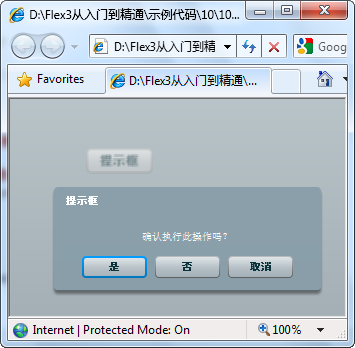6.4 设置提示框的文本大小

```<?xml version="1.0" encoding="utf-8"?>
<!-- 设置对话框的样式 -->
<mx:Style>
{
font-size:14px;
}
</mx:Style>
<mx:Script>
<![CDATA[
// 按钮单击事件
private function Btn_Click():void
{
// 设置对话框的宽度和高度
}
]]>
</mx:Script>
<mx:Button click="Btn_Click();" label="警告框"  x="77" y="50" fontSize="12"/>
</mx:Application>```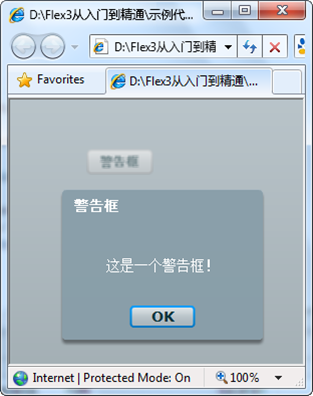6.5 设置提示框的图标

```<?xml version="1.0" encoding="utf-8"?>
<mx:Script>
<![CDATA[
// 嵌入图片资源
[Bindable]
private function Btn_Click():void
{
}
]]>
</mx:Script>
<mx:Button click="Btn_Click();" label="警告框"  x="77" y="50" fontSize="12"/>
</mx:Application>```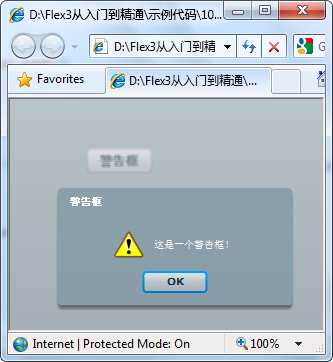7.1创建ProgressBar控件

```<?xml version="1.0" encoding="utf-8"?>
<mx:Script>
<![CDATA[
{
// 加载图片
}
]]>
</mx:Script>
<mx:Button x="10" y="10" label="加载图片" fontSize="12" click="LoadImage()"/>
<mx:ProgressBar x="10" y="42" source="img"/>
<mx:Image id="img" x="10" y="78"/>
</mx:Application>```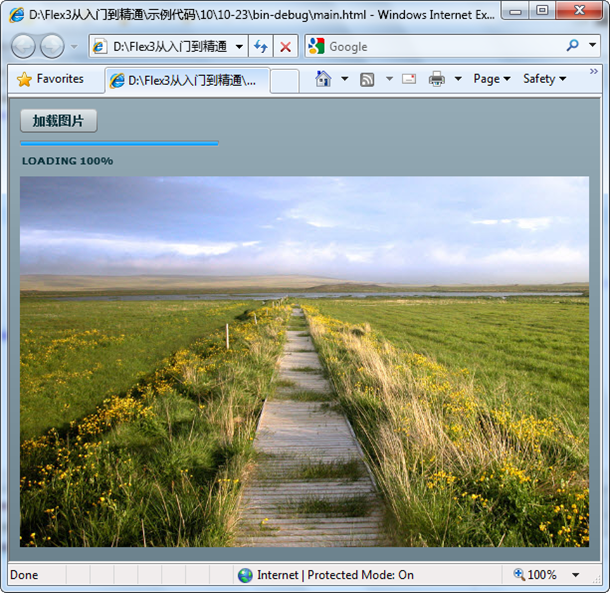7.2 设置进度条的标签

?%1：已经加载的字节数。

?%2：字节总数。

?%3：加载的百分比。

?%%：百分比符号（%）。

```<?xml version="1.0" encoding="utf-8"?>
<mx:Script>
<![CDATA[
{
// 加载图片
}
private function InitProgBar():void
{
// 设置进度条文字说明
progBar.label = "图片大小：%2 字节，已加载： %1 字节 , 加载百分比：%3%%";
}
]]>
</mx:Script>
<mx:Button x="10" y="10" label="加载图片" fontSize="12" click="LoadImage()"/>
<mx:ProgressBar id="progBar" x="10" y="42" source="img" creationComplete="InitProgBar()"/>
<mx:Image id="img" x="10" y="78"/>
</mx:Application>```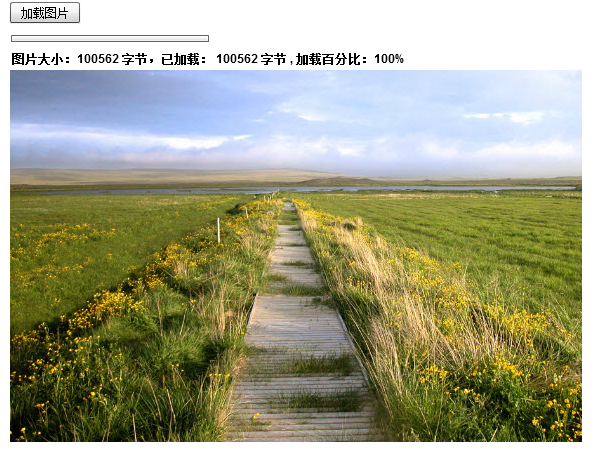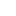喜欢 | 0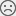不喜欢 | 0

JAVA工程师交流群 +加入

WEB/H5前端交流群 +加入•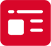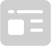索取资料
•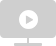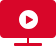答疑解惑
•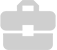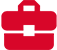技术交流
•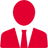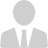职业测评
•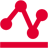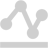面试技巧
•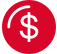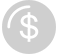高薪秘笈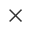Java全栈开发
WEB前端+H5沪公网安备 31011502005948号    ICP许可  沪B2-20190160